A thermodynamic system undergoes cyclic process ABCDA as shown in figure. The work done by the system in the cycle is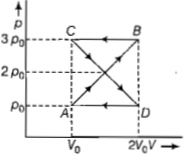(a) ρoV

(b) 2ρoVo

(c) ρoVo/2

(d)zero

Complete Question Bank + Test Series
Complete Question Bank

Difficulty Level:

If Q, E and W denote respectively the heat added, change in internal energy and the work done in a closed cyclic process, then

(a) W=0                                  (b) Q=W=0

(c) E=0                                   (d) Q=0

Complete Question Bank + Test Series
Complete Question Bank

Difficulty Level:

In a cyclic process, the internal energy of the gas -

(1) Increases

(2) Decreases

(3) Remains constant

(4) Becomes zero

Complete Question Bank + Test Series
Complete Question Bank

Difficulty Level:

A thermodynamic system is taken through the cycle PQRSP process. The net work done by the system is -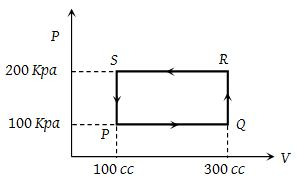(1) 20 J

(2) – 20 J

(3) 400 J

(4) – 374 J

Complete Question Bank + Test Series
Complete Question Bank

Difficulty Level:

An ideal gas is taken around ABCA as shown in the above P-V diagram. The work done during a cycle is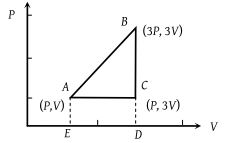(1) 2PV

(2) PV

(3) 1/2PV

(4) Zero

Complete Question Bank + Test Series
Complete Question Bank

Difficulty Level:

An ideal gas is taken through the cycle ABCA, as shown in the figure. If the net heat supplied to the gas in the cycle is 5 J, the work done by the gas in the process CA is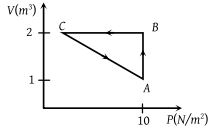(1) – 5 J

(2) – 10 J

(3) – 15 J

(4) – 20 J

Complete Question Bank + Test Series
Complete Question Bank

Difficulty Level:

An ideal gas is taken around the cycle ABCA as shown in the P-V diagram. The net work done by the gas during the cycle is equal to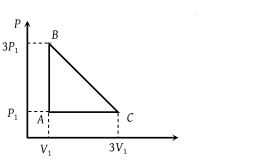(1) 12 P1V1

(2) 6 P1V1

(3) 3 P1V1

(4) 2 P1V1

Complete Question Bank + Test Series
Complete Question Bank

Difficulty Level:

Heat energy absorbed by a system in going through a cyclic process shown in figure is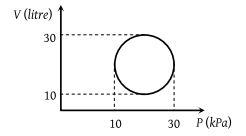(1) 107 π J

(2) 104 π J

(3) 102π J

(4) 10–3π J

Complete Question Bank + Test Series
Complete Question Bank

Difficulty Level:

In the diagrams (i) to (iv) , variation of volume with changing pressure is shown. A gas is taken along the path ABCD. The change in internal energy of the gas will be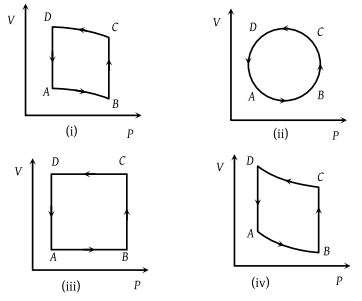(1) Positive in all cases (i) to (iv)

(2) Positive in cases (i), (ii) and (iii) but zero in (iv) case

(3) Negative in cases (i), (ii) and (iii) but zero in (iv) case

(4) Zero in all four cases

Complete Question Bank + Test Series
Complete Question Bank

Difficulty Level:

Carbon monoxide is carried around a closed cycle abc in which bc is an isothermal process as shown in the figure. The gas absorbs 7000 J of heat as its temperature increases from 300 K to 1000 K in going from a to b. The quantity of heat rejected by the gas during the process ca is -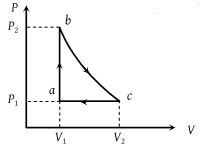(1) 4200 J

(2) 5000 J

(3) 9000 J

(4) 9800 J